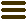The Software Design Metrics tool for the UMLMeasuring the Size and Complexity of UML Sequence Diagrams

October 25, 2012, Jürgen Wüst. Category: Tips & Tricks

Almost all of the UML metrics that SDMetrics calculates “out of the box” are model-centric, not diagram-centric. For example, class metrics count all attributes, associations, operations, etc. of classes. Whether these elements – or the classes themselves – appear on any diagrams does not matter. They are part of the UML model, and that’s what counts.

For practical purposes, diagram-centric metrics are interesting, too. We can use measures of diagram size and complexity to identify large diagrams that could be split up, or select diagrams for design reviews and inspections.

Diagram specific metrics are often difficult to obtain (see this earlier post). An exception here are diagram types that have a corresponding model element in the UML. For example, a statechart diagram directly represents a UML StateMachine model element. Typically, there is only one diagram per StateMachine, and the diagram is usually complete: it shows all the states and transitions of the StateMachine. It is straightforward to define StateMachine metrics that count the states, transitions, regions, etc. of the StateMachine. These metrics then also quantify the size and complexity of the statechart diagram. Similarly, an activity diagram represents a UML Activity model element. By counting the actions, nodes, and flows of an Activity, we effectively obtain size and complexity metrics for activity diagrams. SDMetrics ships with a bunch of StateMachine and Activity metrics, which could be considered diagram-centric in that way.

What about sequence diagrams? A sequence diagram represents a UML Interaction model element, but SDMetrics does not have any Interaction metrics yet. In the following, we’ll take a shot at defining a basic set of simple size and complexity measures for UML 2.x sequence diagrams.

The two most important elements in a sequence diagram are lifelines and messages. A UML Interaction owns all of its lifelines and messages. SDMetrics extracts these into attributes “lifelines” and “messages” (cf. the list of attributes for model element type “interaction”). With that knowledge, counting the lifelines and messages of a UML Interaction is simple. Add the following SDMetricsML definitions to the metrics definition file:

<metric name="Lifelines" domain="interaction" category="size">
<description>The number of lifelines of the interaction.</description>
<projection relset="lifelines"/>
</metric>

<metric name="Messages" domain="interaction" category="complexity">
<description>The number of messages of the interaction.</description>
<projection relset="messages" />
</metric>

As a rule of thumb, metrics counting the nodes of a graph measure size, and metrics counting the edges measure complexity (see this paper on property-based software measurement). If we consider lifelines to be the nodes of a sequence diagrams, and messages to be edges, we can classify “Lifelines” as a size metric and “Messages” as a complexity metric.

Depending on our measurement needs at hand, we might want to count only certain types of messages. For example, to identify sequence diagrams with predominantly asynchronous message passing, we would only count asynchronous messages. The “message” element type has an attribute “sort” that indicates the type. For asynchronous messages, the “sort” attribute has the value “asynchCall”. So we can count the asynchronous messages as follows:

<metric name="AsynchMessages" domain="interaction">
<projection relset="messages" condition="sort='asynchCall'" />
</metric>

In the same way, we could separately count signals, reply messages, create messages, or delete messages. To only count synchronous messages, however, requires a bit of attention. The UML defines the default message sort to be synchronous (“synchCall”). Some XMI exporters do not write out the message sort attribute for synchronous messages, and SDMetrics’ XMI parser does not supply default values either. Therefore, the value of the sort attribute for synchronous messages can be “synchCall” or empty. So the proper condition to identify synchronous messages is sort='' or sort='synchCall'. If we need to check this condition in more than one place (maybe for some custom design rules pertaining to synchronous messages), we can define a helper metric like so:

<metric name="IsSynchMsg" domain="message" internal="true">
<compoundmetric condition="sort='' or sort='synchCall'"/>
</metric>

The value of this conditional compound metric is 1 for synchronous messages, and 0 for all other messages. If our notion of what constitutes synchronous messages changes, we only need to adapt the helper metric. Our metric to count synchronous messages then looks as follows:

<metric name="SynchMessages" domain="interaction">
<projection relset="messages" condition="isSynchMsg=1" />
</metric>

The next important element types in sequence diagrams are combined fragments and interaction operands. Combined fragments can be nested, so we use the subelements procedure to count all fragments directly or indirectly owned by the interaction:

<metric name="CombinedFragments" domain="interaction">
<subelements target="combinedfragment"/>
</metric>

A combined fragment contains one or more interaction operands. To count the interaction operands in the sequence diagram, we take the sum of the number of interaction operands over all combined fragments:

<metric name="InteractionOperands" domain="interaction">
<subelements target="combinedfragment" sum="size(operands)"/>
</metric>

So that’s our first set of simple size and complexity metrics for UML sequence diagrams. In a subsequent post, I’ll tackle some more advanced measures or design rules, maybe “self/recursive message calls” (how often do lifelines send messages to themselves), the nesting level of combined fragments (don’t overdo it), or the “height” of sequence diagram in terms of the maximum number of messages on a lifeline. We’ll see.